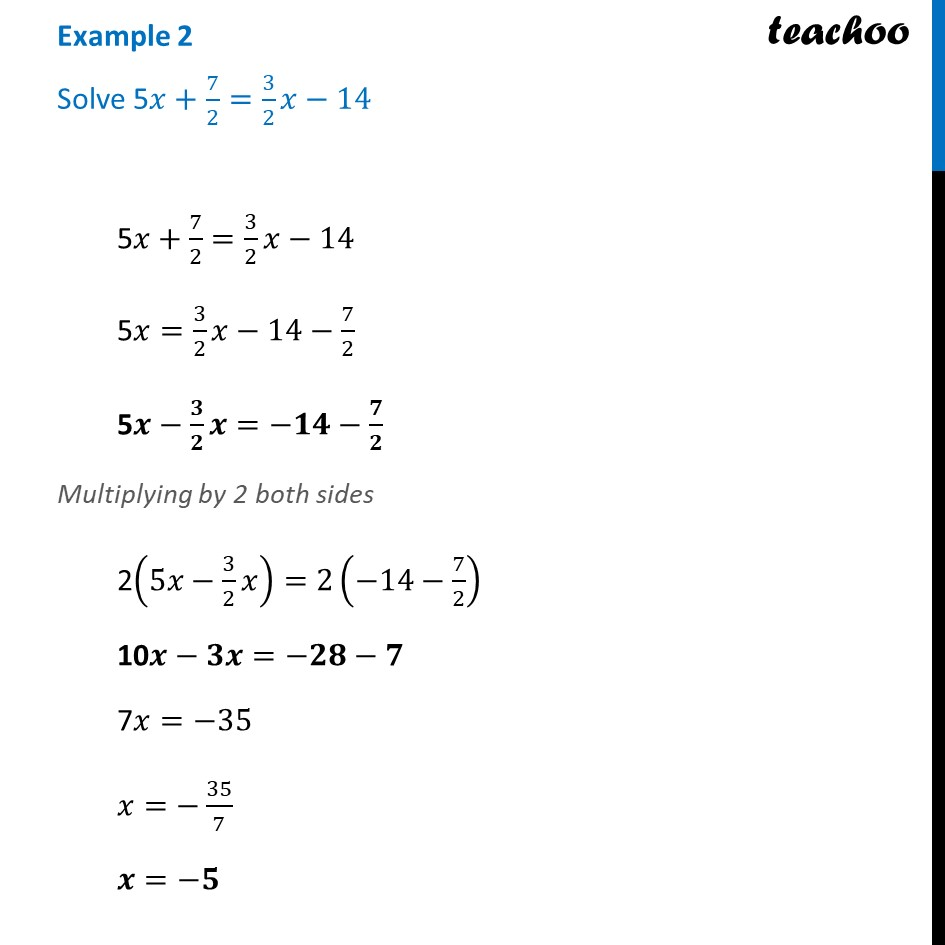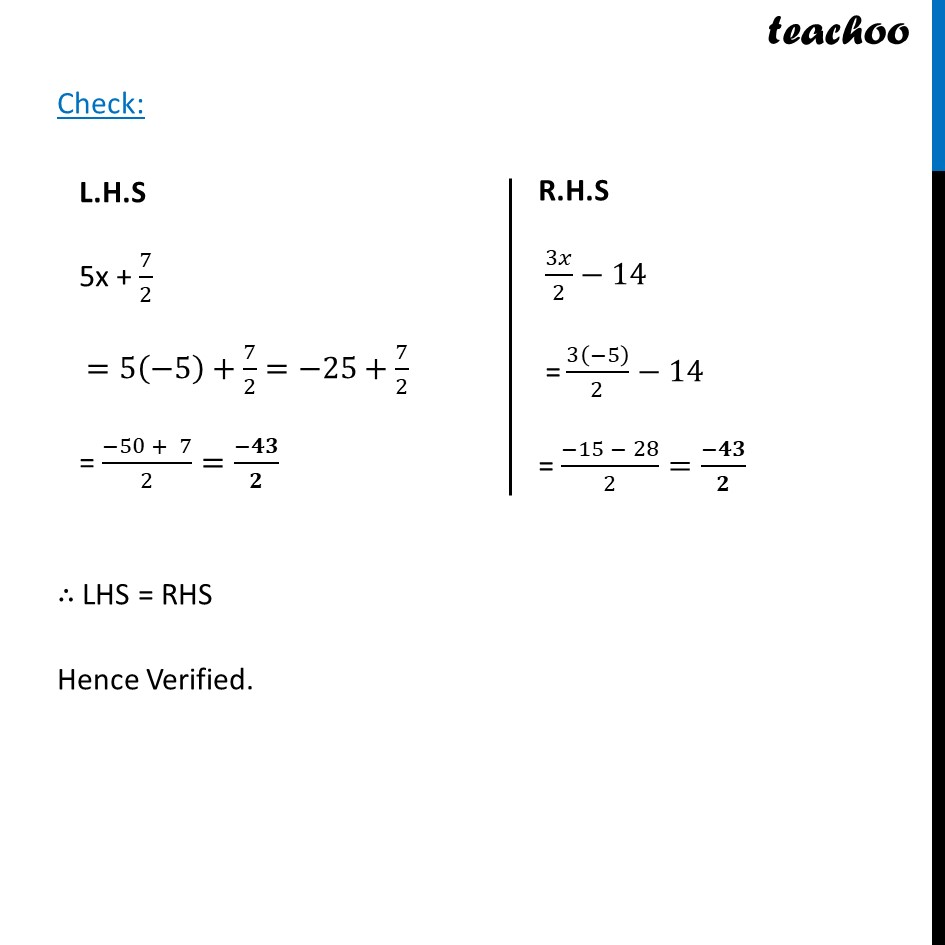Examples

Chapter 2 Class 8 Linear Equations in One Variable
Serial order wiseLearn in your speed, with individual attention - Teachoo Maths 1-on-1 Class

### Transcript

Example 2 Solve 5𝑥+ 7/2=3/2 𝑥−145𝑥+ 7/2=3/2 𝑥−14 5𝑥=3/2 𝑥−14−7/2 5𝒙−𝟑/𝟐 𝒙=−𝟏𝟒−𝟕/𝟐 Multiplying by 2 both sides 2(5𝑥−3/2 𝑥)=2(−14−7/2) 10𝒙−𝟑𝒙=−𝟐𝟖−𝟕 7𝑥=−35 𝑥=−35/7 𝒙=−𝟓 L.H.S 5x + 7/2 =5(−5)+7/2=−25+7/2 = (−50 + 7)/2=(−𝟒𝟑)/𝟐 R.H.S 3𝑥/2−14" " " =" 3(−5)/2−14 = (−15 − 28)/2=(−𝟒𝟑)/𝟐 Check: ∴ LHS = RHS Hence Verified.